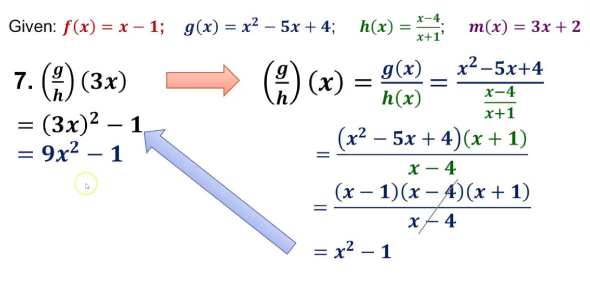# Ultimate General Mathematics Quiz

15 Questions | Total Attempts: 166SettingsMathematics quiz, Let’s find out how much you know with this awesome quiz!

Related Topics
• 1.
Where would you see the use of Piezoelectric effect?
• A.

CT Scan

• B.

MRI

• C.

Ultrasonography

• D.

ECG

• 2.
If 256 x 0.5 = 1024 ≈ Z x 4, what is Z?
• A.

62

• B.

32

• C.

15

• D.

45

• 3.
What is the value of 92.5% of 550?
• A.

505

• B.

515.75

• C.

512.33

• D.

508.75

• 4.
Calculate the compound interest of \$4000 at 5% p.a. after 3 years?
• A.

675

• B.

625

• C.

725

• D.

630.5

• 5.
What is 30% of 40% of 2/5 of 2500?
• A.

180

• B.

160

• C.

120

• D.

190

• 6.
If the average of A, B, C and D, 4 consecutive even nos. is 65, what is A x B?
• A.

3736

• B.

4368

• C.

4216

• D.

4688

• 7.
What factor of 72 is missing in these numbers: 1, 2, 4, 8, 9, 18, 72?
• A.

33

• B.

12

• C.

36

• D.

24

• 8.
What will be the angle between 2 hands of a clock at 7:30AM?
• A.

45 degrees

• B.

60 degrees

• C.

25 degrees

• D.

30 degrees

• 9.
If 3 days before yesterday was Saturday, what'll be 5 days after tomorrow?
• A.

Wednesday

• B.

Friday

• C.

Monday

• D.

Tuesday

• 10.
If P's wage decreases by 20% and then increases by 10%, what's the % change?
• A.

15% increase

• B.

10% increase

• C.

12% reduction

• D.

10% reduction

• 11.
If the sides of a rectangle decrease by 30%, how much will its area decrease?
• A.

55%

• B.

30%

• C.

51%

• D.

25%

• 12.
If 4th issue of a weekly releases in 7th Jan 1885, when'll the 40th issue release?
• A.

16 Sept

• B.

16-Jun

• C.

16-Jul

• D.

16-May

• 13.
For a circle, the circumference - radius = 74cm, what is its area?
• A.

616 sq cm

• B.

312 sq cm

• C.

466 sq cm

• D.

586 sq cm

• 14.
What will be the volume of a cylinder with radius 5 cm and height 10 cm?
• A.

885.5 cm^3

• B.

785.5 cm^3

• C.

755.5 cm^3

• D.

995.5 cm^3

• 15.
What is the volume of a brick 40 cm by 15 cm by 10 cm?
• A.

6200 cm^3

• B.

1600 cm^3

• C.

600 cm^3

• D.

6000 cm^3